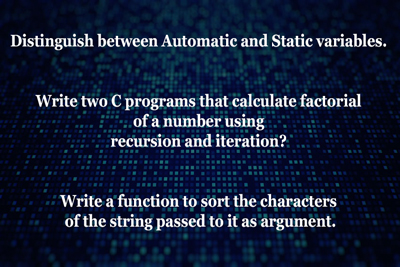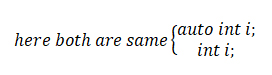auto and static variables, Sort the character, factorial of a Number
20-04-2020    102 times### Distinguish between Automatic and Static variables. Write two C programs that calculate factorial of a number using recursion and iteration? Write a function to sort the characters of the string passed to it as argument.

Distinguish between Automatic and Static variables.

Automatic variables are declared by the keyword “auto” or if we are not declared a variable without any storage class keyword then it assign as an “automatic variables”. Automatic variables are store in t RAM or primary memories with initialized by a garbage value. These variables are newly declared when a function called.

Declaration of an automatic variable.Static variables are declared by the keyword “static”. Static variables are store in t RAM or primary memories with initialized by 0. These variables are declared only once in a program.

Declaration of a static variable.
static int i;

Write two C programs that calculate factorial of a number using recursion and iteration?

Using Recursion

```void main()
{
int f=5,res=0;
res=fact(f);
printf("Factorial of %d is %d",f,res);
}

int fact(int f)
{
int r=1;
if(f==0)
{
return r;
}
else
{
r=f*fact(f-1);
}

}

```

Using Iteration

```void main()
{
int f=5,res=0;
res=fact(f);
printf("Factorial of %d is %d",f,res);
}

int fact(int f)
{
int r=1;
for(;f>0;f--)
{
r = r * f;
}
return r;
}
```

Write a function to sort the characters of the string passed to it as the argument.```#include<string.h>

char *charsort(char *);
void main()
{
char str,ch;
char *s;
int i,j,len;
clrscr();
printf("Enter a string");
gets(str);
s=charsort(str);

printf("\n Sorted String :");
puts(s);
}
char *charsort(char *s)
{
char ch;
int len,i,j;
len=strlen(s);
for(i=0;i<len-1;i++)
{
for(j=i+1;j<len;j++)
{
if(*(s+i)>*(s+j))
{
ch=*(s+i);
*(s+i)=*(s+j);
*(s+j)=ch;
}
}
}
return s;
}
```